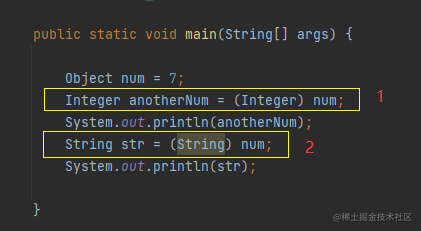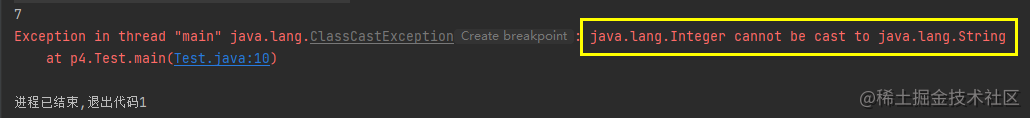# Java泛型T、E、K、V、N、?和Object区别和含义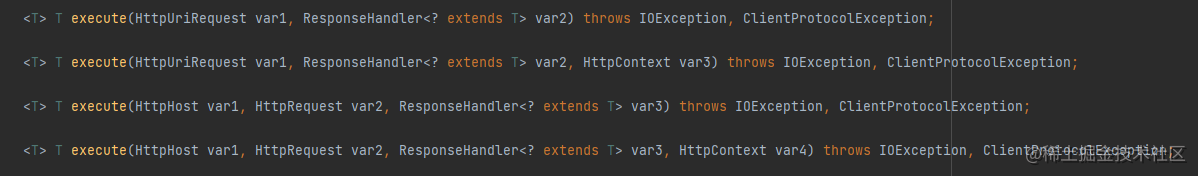## 什么是泛型

Java 泛型（generics）是 JDK 5 中引入的一个新特性, 泛型提供了编译时类型安全检测机制，该机制允许程序员在编译时检测到非法的类型。

``````public class Result implements Serializable {

// 响应码
Integer code;

// 是否成功
Boolean success;

// 返回体数据
User user;

public Result(Integer code, Boolean success, User user) {
this.code = code;
this.success = success;
this.user = user;
}

@Override
public String toString() {
return "Result{" +
"code=" + code +
", success=" + success +
", user=" + user +
'}';
}

public static void main(String[] args) {

User user = new User(1, "Tony");
Result result = new Result(200, true, user);
System.out.println(result);

}

}

class User implements Serializable {

Integer id;

String name;

public User(Integer id, String name) {
this.id = id;
this.name = name;

}

@Override
public String toString() {
return "User{" +
"id=" + id +
", name='" + name + '\'' +
'}';
}
}

``````Result{code=200, success=true, user=User{id=1, name='Tony'}}

``````public class Result<T> implements Serializable {

// 响应码
Integer code;

// 是否成功
Boolean success;

// 返回体数据
T data;

public Result(Integer code, Boolean success, T data) {
this.code = code;
this.success = success;
this.data = data;
}

@Override
public String toString() {
return "Result{" +
"code=" + code +
", success=" + success +
", data=" + data +
'}';
}

public static void main(String[] args) {

User user = new User(1, "Tony");
Result<User> resultUser = new Result<>(200, true, user);
System.out.println(resultUser);
Phone phone = new Phone(999.99, "Yellow");
Result<Phone> resultPhone = new Result<>(200, true, phone);
System.out.println(resultPhone);

}

}

class User implements Serializable {

Integer id;

String name;

public User(Integer id, String name) {
this.id = id;
this.name = name;

}

@Override
public String toString() {
return "User{" +
"id=" + id +
", name='" + name + '\'' +
'}';
}
}

class Phone implements Serializable {

Double price;

String color;

@Override
public String toString() {
return "Phone{" +
"price=" + price +
", color='" + color + '\'' +
'}';
}

public Phone(Double price, String color) {
this.price = price;
this.color = color;
}
}

``````Result{code=200, success=true, data=User{id=1, name='Tony'}}
Result{code=200, success=true, data=Phone{price=999.99, color='Yellow'}}

## 泛型方法

### 语法规则

• 所有泛型方法声明都有一个类型参数声明部分（由尖括号分隔），该类型参数声明部分在方法返回类型之前

``````private static <E> void printArray(E[] inputArray)

• 每一个类型参数声明部分包含一个或多个类型参数，参数间用逗号隔开。一个泛型参数，也被称为一个类型变量，是用于指定一个泛型类型名称的标识符。

``````private static <E,T> void printArray(E[] inputArray, T data)

• 类型参数能被用来声明返回值类型，并且能作为泛型方法得到的实际参数类型的占位符

• 泛型方法体的声明和其他方法一样。注意类型参数只能代表引用型类型，不能是原始类型(int double char等)

### 泛型标记符

• E Element 集合元素
• T Type Java类
• K Key 键
• V Value 值
• N Number 数值类型
• ？ 表示不确定的Java类型

``````public class TFunction {

public static void main(String[] args) {

// 创建各种类型的数组
Integer[] intArray = {1, 2, 3, 4, 5};
Double[] doubleArray = {1.1, 2.2, 3.3, 4.4};
Character[] charArray = {'H', 'E', 'L', 'L', 'O'};

System.out.println("整型数组元素为:");
printArray(intArray); // 传递一个整型数组

System.out.println("\n双精度型数组元素为:");
printArray(doubleArray); // 传递一个双精度型数组

System.out.println("\n字符型数组元素为:");
printArray(charArray); // 传递一个字符型数组

}

// 泛型方法
private static <E> void printArray(E[] inputArray) {

// 遍历打印数组
Arrays.stream(inputArray).forEach(e -> {
System.out.printf("%s ", e);
});
System.out.println();

}

}

## 类型通配符

### ？

``````public class GenericTest {

public static void main(String[] args) {
List<String> name = new ArrayList<String>();
List<Integer> age = new ArrayList<Integer>();
List<Number> number = new ArrayList<Number>();

getData(name);
getData(age);
getData(number);

}

public static void getData(List<?> data) {
System.out.println("data :" + data.get(0));
}
}

``````data :icon
data :18
data :314

### ？extends T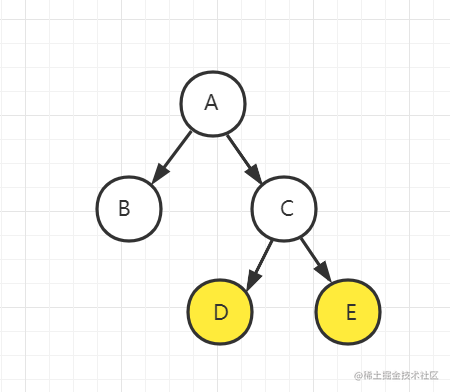### ? super T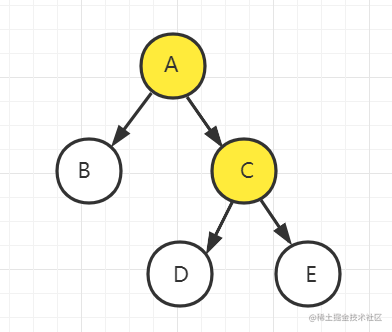## T 和 ？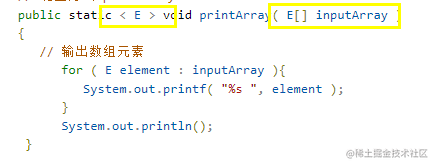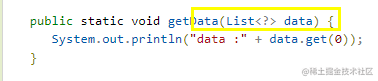## T 和 Object

Object是所有类的父类，在编码过程中就难免出现类型转化问题，且在编译阶段不会报错，到了运行阶段才暴露问题，大大降低了程序的安全性健壮性

• 向上转型 用父类声明一个子类对象 例如：Animal是Cat的父类，在声明时我们这么写：
``````  Animal cat = new Cat();

• 向下转型

将父类对象强转为其子类实例:

``````Animal cat = new Cat();
Cat anotherCat = (Cat) cat;